1. ##Eaty system

I posted this in FF but seem like not much ppl interested. Anyway i want to see anyone interested in making this into EA.

I had developed this system for sometime. It is base on H4 time frame.

For start let make it short. If anyone interested with it I only write more. As my English suck so I make it into point form and graphically for easy understanding. Hope there are no typo. : )

Time frame: H4
Pair: GBPJPY (This pair perform well.)
Indicator: RSI (3), RSI (4), MACD (7, 13, 1)

* RSI (3) and RSI (4) to cross above 50.
* MACD (7, 13, 1) has to be in green color.
* After 3 signal confirmed, the next bar start we put 3 pending buy orders.

1.Entry = High + 16pips + Spread, TP = 50, SL (Pls read Stop Loss written below.)
2.Entry = High + 16pips + Spread, TP = 100, SL (Pls read Stop Loss written below.)
3.Entry = High + 16pips + Spread, TP = freely until the SL hit, SL (Pls read Stop Loss written below.)
For non GJ your TP will need to change as below:
TP1 = 15
Tp2 = 30
TP3 = freely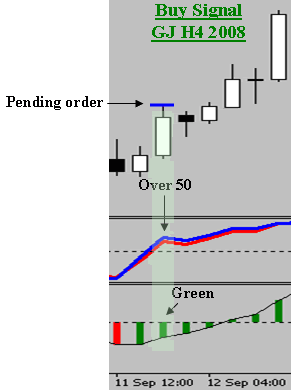Sell Enter:

* RSI (3) and RSI (4) to cross below 50.
* MACD (7, 13, 1) has to be in red color.
* After 3 signal confirmed, the next bar start we put 3 pending sell orders.

1.Entry = High - 16pips - Spread, TP = 50, SL (Pls read Stop Loss written below.)
2.Entry = High - 16pips - Spread, TP = 100, SL (Pls read Stop Loss written below.)
3.Entry = High - 16pips - Spread, TP = freely until the SL hit, SL (Pls read Stop Loss written below.)
For non GJ your TP will need to change as below:
TP1 = 15
Tp2 = 30
TP3 = freely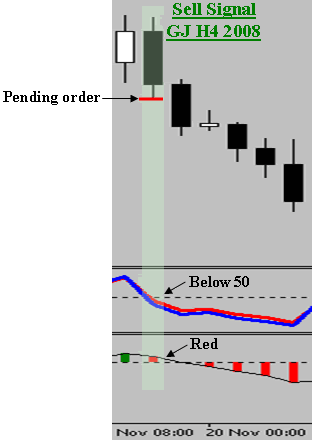Stop Loss (SL):
Case1: Set at the opposite entry.

Case2: If the H4 bar achieves > 150 pips profit, at the close of that H4 bar set our SL to 50 pips.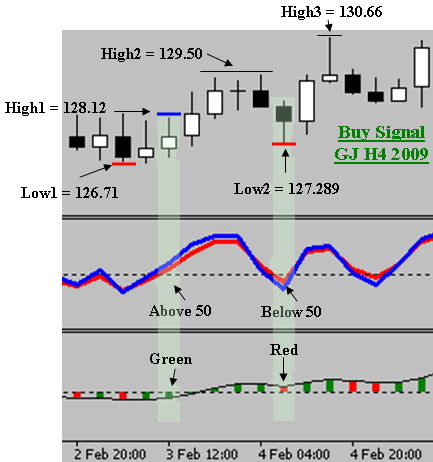SL Case1 + SL Case2: Let look at the High1 for pending buy order.

Buy entry = 128.12 (High1) + 16 pips + 7 pips (spread) = 128.35
SL = 126.71 (Low1) – 16 pips – 0 (Spread) = 126.55
High2 – Entry = 129.50 - 128.35 = 115 pips

Trade3 (T3) TP = not yet hit cos it hasn’t hit our SL, SL = 126.55
So T3 continue running. Until SL rules apply.

Sell entry = New SL (Base on Case1)

So a Pending sell order and new SL for T3. Open until it close. For this case it move up to High3. SL case2 rules apply.
New SL = 50 pips (Base on case2, 130.66 (High3) – 128.35 (Entry) = 231).

Let it run until new SL rules apply. In this case it close at our SL.

T1 TP = 50
T2 TP = 100
T3 TP = 50
Total profit = 50 + 100 + 50 = 200 pips

Case3:

* RSI (3) form a new low above 50 for buy.
* RSI (3) form a new high below 50 for sell.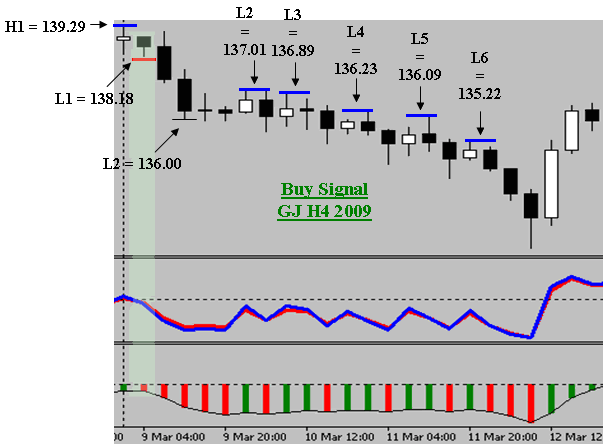Case3.b: This pic show sell order with SL case3

Sell entry =138.18 (L1) - 16 pips - 0 pips (spread) = 138.02
SL = buy entry = 139.29(H1) + 16 pips + 7 pips (Spread) = 139.52
Entry – L2 = 138.02 – 136.00 = 202
16 pips + 7 spread = 23 pips

Trade3 (T3) TP = not yet hit cos it hasn’t hit our SL, SL = 139.52

SL progress
139.52 (Case1)
-> 138.02(Entry) – 50 pips = 137.52 (Case2)
-> 137.01(L2) + 23 pips = 137.24 (Case3)
-> 136.89(L3) + 23 pips = 137.12 (Case3)
-> 136.23 (L4)+ 23 pips = 136.46 (Case3)
-> 136.09 (L5) + 23 pips = 136.32 (Case3)
-> 135.22 (L6) + 23 pips = 135.45 (Case3)

Trade3 (T3) TP = 138.02 – 135.45 = 257

Total profit = 50 +100 +257 = 407

Case4:

* RSI (3) move below 50 for buy.
* RSI (3) move above 50 for sell.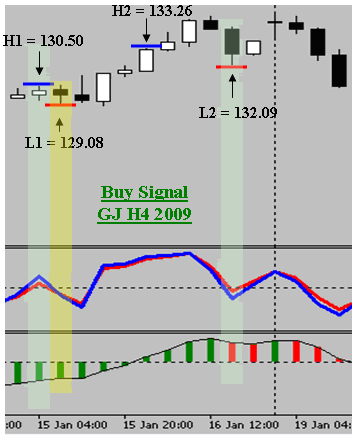Case4.a: This pic show sell order with SL case4

Buy entry =130.50 (H1) + 16 pips + 7 pips (spread) = 130.73

SL = For this case we don’t have SL at start cos previous sell had close. So we don’t set SL until SL rules apply. RSI (3) below 50 apply after our buy entry signal. So we will use that as a SL. SL = L1 – 16 pips = 128.92

Entry – H2 = 130.73 – 133.26 = 253

SL progress
128.92 ->
-> 130.73 (Entry) + 50 pips = 131.23(Case2)
-> 132.09 (L2) – 16 pips = 131.93 (Case4)

Trade3 (T3) TP = 130.73 – 131.93 = 120
Total profit = 50 +100 +120 = 270

*** No trade when MACD(7, 13, 1) < 30 Or >-30.
In profit set BE.
Example:

1. 01 Jun 09 GBYJPY Buy order. (390 pips)
Part 1
Part 2
Part 3
Part 4

2. 02 Jun 09 GBYJPY Sell order. (88 pips)
Part 1
Part 2
Part 3Reply With Quote

2. ##Hope after you see the backtest you feel interested

Due to i stop this method i don't hv new real time back test result. So i post my old backtest result.

I stop this method because it is super tiring if u trade every 4 hour. I set my alarm every 4 hour wake up n trade. Cos me mental n physical tired. If with ea it will be more easier.

I use markativa to trade this method. So you will see 2 type of print screen. 1 is history 1 is from real time. Both are same it is my account history.

The trade are more but the system i explain only 3 trade. So just ignored the extra trade i traded. We can trade 3 as i explain above. As old data that i had is only these. Anyway the extra trade can see the potential.

Sorry for the messy look on the result as i cut the result 1 by 1 from my old journal. I cant post many result 1 in post as it go messy.

Both mth is only trading GJ
Sep'08 : +8673 pips
Oct'08 : +321 pips
This system can be improve with the help with ea. Such as, when in profit Break even. When i trade live i log in every 4 hour so i nv BE. Result is base on older version of my system. Now i improve the system with lower TP,RSI and SL method . So the result is better. Hope you like it. Anyway ty in advance.

01 Sep 08 Result Total Net profit : +1169 pipsReply With Quote

3. ##03 Sep 08 Result Total Net profit : +114 pips
04 Sep 08 Result Total Net profit : +950 pipsReply With Quote

4. ##08 Sep 08 Result Total Net profit : +2210 pipsReply With Quote

5. ##12 Sep 08 Result Total Net profit : +753 pips
15 Sep 08 Result Total Net profit : -9 pipsReply With Quote

6. ##17 Sep 08 Result Total Net profit : +1272 pips
26 Sep 08 Result Total Net profit : +602 pipsReply With Quote

7. ##29 Sep 08 Result Total Net profit : +727 pips
30 Sep 08 Result Total Net profit : +885 pipsReply With Quote

8. ##01 Oct 08 Result Total Net profit : -648 pips
03 Sep 08 Result Total Net profit : +612 pipsReply With Quote

9. ##03 Oct 08 Result Total Net profit : +352 pips
06 Oct 08 Result (Early morning) Total Net profit : -2690 pipsReply With Quote

10. ##6 Oct 08 Result (Late night) Total Net profit : +2533 pips
7 Oct 08 Result Total Net profit : +864 pipsReply With Quote

####Posting Permissions

• You may not post new threads
• You may not post replies
• You may not post attachments
• You may not edit your posts
•